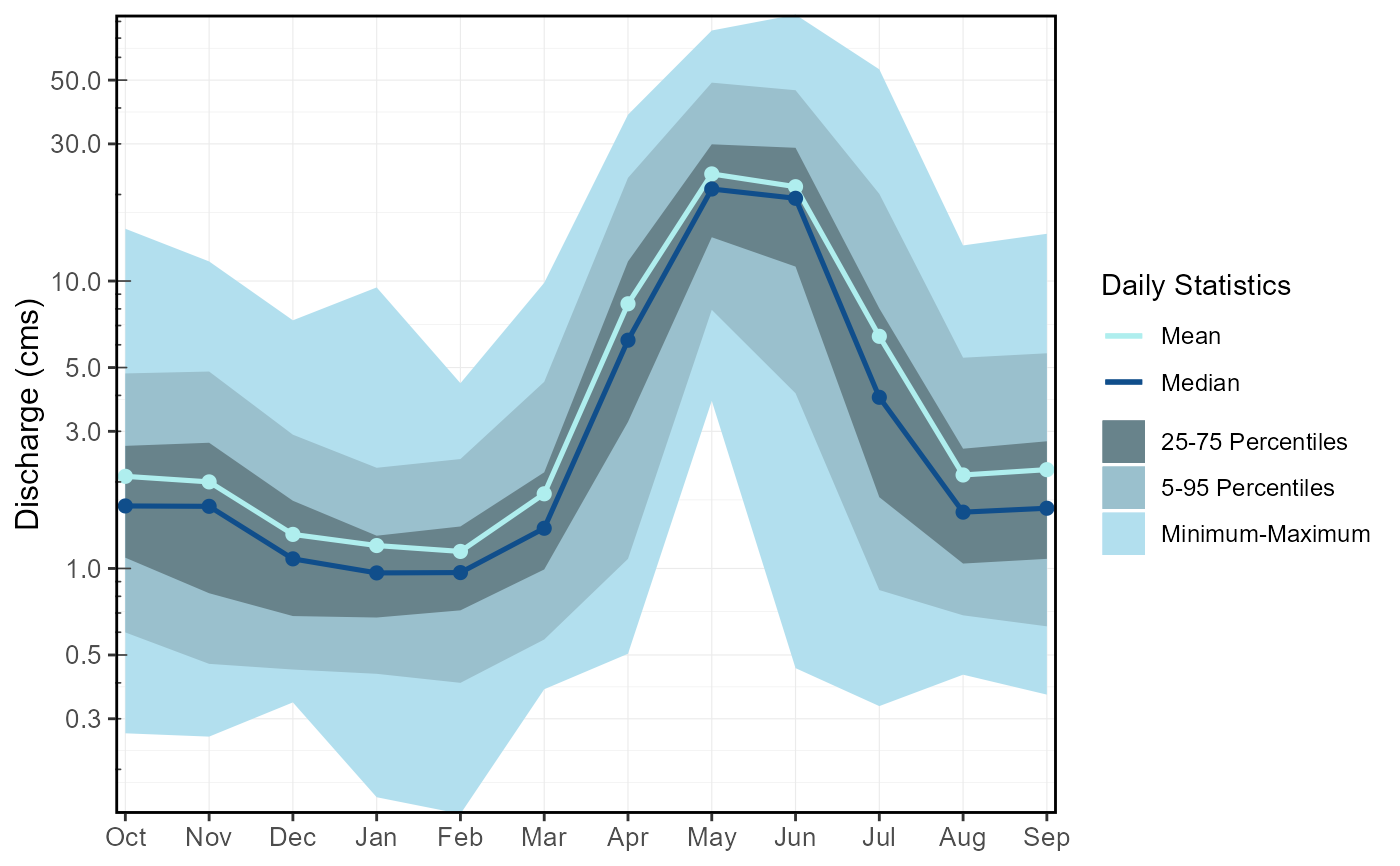Plots the long-term mean, median, maximum, minimum, and percentiles of daily flow values for over all months and all data (Long-term) from a daily streamflow data set. Calculates statistics from all values, unless specified. The Maximum-Minimum band can be removed using the include_extremes argument and the percentile bands can be customized using the inner_percentiles and outer_percentiles arguments. Data calculated using the calc_longterm_daily_stats() function. Returns a list of plots.

plot_longterm_daily_stats(
data,
dates = Date,
values = Value,
groups = STATION_NUMBER,
station_number,
roll_days = 1,
roll_align = "right",
water_year_start = 1,
start_year,
end_year,
exclude_years,
complete_years = FALSE,
ignore_missing = FALSE,
include_extremes = TRUE,
inner_percentiles = c(25, 75),
outer_percentiles = c(5, 95),
add_year,
log_discharge = TRUE,
include_title = FALSE
)

## Arguments

data Data frame of daily data that contains columns of dates, flow values, and (optional) groups (e.g. station numbers). Leave blank if using station_number argument. Name of column in data that contains dates formatted YYYY-MM-DD. Only required if dates column name is not 'Date' (default). Leave blank if using station_number argument. Name of column in data that contains numeric flow values, in units of cubic metres per second. Only required if values column name is not 'Value' (default). Leave blank if using station_number argument. Name of column in data that contains unique identifiers for different data sets, if applicable. Only required if groups column name is not 'STATION_NUMBER'. Function will automatically group by a column named 'STATION_NUMBER' if present. Remove the 'STATION_NUMBER' column beforehand to remove this grouping. Leave blank if using station_number argument. Character string vector of seven digit Water Survey of Canada station numbers (e.g. "08NM116") of which to extract daily streamflow data from a HYDAT database. Requires tidyhydat package and a HYDAT database. Leave blank if using data argument. Numeric value of the number of days to apply a rolling mean. Default 1. Character string identifying the direction of the rolling mean from the specified date, either by the first ('left'), last ('right'), or middle ('center') day of the rolling n-day group of observations. Default 'right'. Numeric value indicating the month (1 through 12) of the start of water year for analysis. Default 1. Numeric value of the first year to consider for analysis. Leave blank to use the first year of the source data. Numeric value of the last year to consider for analysis. Leave blank to use the last year of the source data. Numeric vector of years to exclude from analysis. Leave blank to include all years. Logical values indicating whether to include only years with complete data in analysis. Default FALSE. Logical value indicating whether dates with missing values should be included in the calculation. If TRUE then a statistic will be calculated regardless of missing dates. If FALSE then only those statistics from time periods with no missing dates will be returned. Default FALSE. Logical value to indicate plotting a ribbon with the range of daily minimum and maximum flows. Default TRUE. Numeric vector of two percentile values indicating the lower and upper limits of the inner percentiles ribbon for plotting. Default c(25,75), set to NULL for no inner ribbon. Numeric vector of two percentile values indicating the lower and upper limits of the outer percentiles ribbon for plotting. Default c(5,95), set to NULL for no outer ribbon. Numeric value indicating a year of daily flows to add to the daily statistics plot. Leave blank for no years. Logical value to indicate plotting the discharge axis (Y-axis) on a logarithmic scale. Default FALSE. Logical value to indicate adding the group/station number to the plot, if provided. Default FALSE.

## Value

A list of ggplot2 objects with the following for each station provided:

Long-term_Monthly_Statistics

a plot that contains long-term flow statistics

Default plots on each object:
Monthly Mean

mean of all annual monthly means for a given month over all years

Monthly Median

median of all annual monthly means for a given month over all years

25-75 Percentiles Range

a ribbon showing the range of data between the monthly 25th and 75th percentiles

5-95 Percentiles Range

a ribbon showing the range of data between the monthly 5th and 95th percentiles

Max-Min Range

a ribbon showing the range of data between the monthly minimum and maximums

## See also

calc_longterm_daily_stats

## Examples

# Run if HYDAT database has been downloaded (using tidyhydat::download_hydat())
if (file.exists(tidyhydat::hy_downloaded_db())) {

# Plot longterm daily statistics using data argument with defaults
flow_data <- tidyhydat::hy_daily_flows(station_number = "08NM116")
plot_longterm_daily_stats(data = flow_data,
start_year = 1980)

# Plot longterm daily statistics for water years starting in October
plot_longterm_daily_stats(station_number = "08NM116",
start_year = 1980,
end_year = 2010,
water_year_start = 10)

}
#> \$Long-term_Daily_Statistics#>